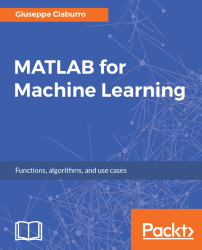•#### MATLAB for Machine Learning#### Overview of this book

MATLAB is the language of choice for many researchers and mathematics experts for machine learning. This book will help you build a foundation in machine learning using MATLAB for beginners. You’ll start by getting your system ready with t he MATLAB environment for machine learning and you’ll see how to easily interact with the Matlab workspace. We’ll then move on to data cleansing, mining and analyzing various data types in machine learning and you’ll see how to display data values on a plot. Next, you’ll get to know about the different types of regression techniques and how to apply them to your data using the MATLAB functions. You’ll understand the basic concepts of neural networks and perform data fitting, pattern recognition, and clustering analysis. Finally, you’ll explore feature selection and extraction techniques for dimensionality reduction for performance improvement. At the end of the book, you will learn to put it all together into real-world cases covering major machine learning algorithms and be comfortable in performing machine learning with MATLAB.
Title PageCreditsForewordwww.PacktPub.comCustomer FeedbackPrefaceFree Chapter
Getting Started with MATLAB Machine LearningImporting and Organizing Data in MATLABFrom Data to Knowledge DiscoveryFinding Relationships between Variables - Regression TechniquesPattern Recognition through Classification AlgorithmsIdentifying Groups of Data Using Clustering MethodsSimulation of Human Thinking - Artificial Neural NetworksImproving the Performance of the Machine Learning Model - Dimensionality ReductionMachine Learning in Practice## Partitioning-based clustering methods - K-means algorithm

K-means clustering is a partitioning method and as anticipated, this method decomposes a dataset into a set of disjoint clusters. Given a dataset, a partitioning method constructs several partitions of this data, with each partition representing a cluster. These methods relocate instances by moving them from one cluster to another, starting from an initial partitioning.

### The K-means algorithm

The K-means algorithm is a clustering algorithm designed in 1967 by MacQueen which allows the dividing of groups of objects into K partitions based on their attributes. It is a variation of the expectation-maximization (EM) algorithm, whose goal is to determine the K data groups generated by Gaussian distributions. The K-means algorithm differs in the method used for calculating the Euclidean distance while calculating the distance between each of two data items; EM uses statistical methods.

In K-means, it is assumed that object attributes can be...